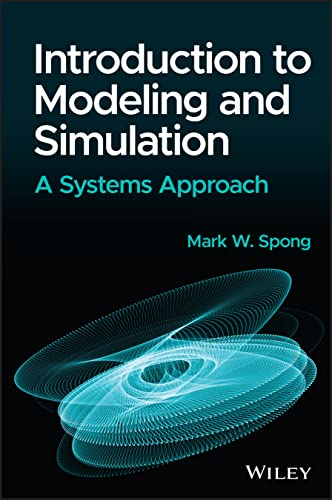# Introduction to Modeling and Simulation: A Systems Approach

• Length: 432 pages
• Edition: 1
• Publisher:
• Publication Date: 2023-02-13
• ISBN-10: 111998288X
• ISBN-13: 9781119982883
Description

Introduction to Modeling and Simulation

An essential introduction to engineering system modeling and simulation from a well-trusted source in engineering and education

This new introductory-level textbook provides thirteen self-contained chapters, each covering an important topic in engineering systems modeling and simulation. The importance of such a topic cannot be overstated; modeling and simulation will only increase in importance in the future as computational resources improve and become more powerful and accessible, and as systems become more complex. This resource is a wonderful mix of practical examples, theoretical concepts, and experimental sessions that ensure a well-rounded education on the topic.

The topics covered in Introduction to Modeling and Simulation are timeless fundamentals that provide the necessary background for further and more advanced study of one or more of the topics. The text includes topics such as linear and nonlinear dynamical systems, continuous-time and discrete-time systems, stability theory, numerical methods for solution of ODEs, PDE models, feedback systems, optimization, regression and more. Each chapter provides an introduction to the topic to familiarize students with the core ideas before delving deeper. The numerous tools and examples help ensure students engage in active learning, acquiring a range of tools for analyzing systems and gaining experience in numerical computation and simulation systems, from an author prized for both his writing and his teaching over the course of his over-40-year career.

Introduction to Modeling and Simulation readers will also find:

• Numerous examples, tools, and programming tips to help clarify points made throughout the textbook, with end-of-chapter problems to further emphasize the material
• As systems become more complex, a chapter devoted to complex networks including small-world and scale-free networks – a unique advancement for textbooks within modeling and simulation
• A complementary website that hosts a complete set of lecture slides, a solution manual for end-of-chapter problems, MATLAB files, and case-study exercises

Introduction to Modeling and Simulation is aimed at undergraduate and first-year graduate engineering students studying systems, in diverse avenues within the field: electrical, mechanical, mathematics, aerospace, bioengineering, physics, and civil and environmental engineering. It may also be of interest to those in mathematical modeling courses, as it provides in-depth material on MATLAB simulation and contains appendices with brief reviews of linear algebra, real analysis, and probability theory.